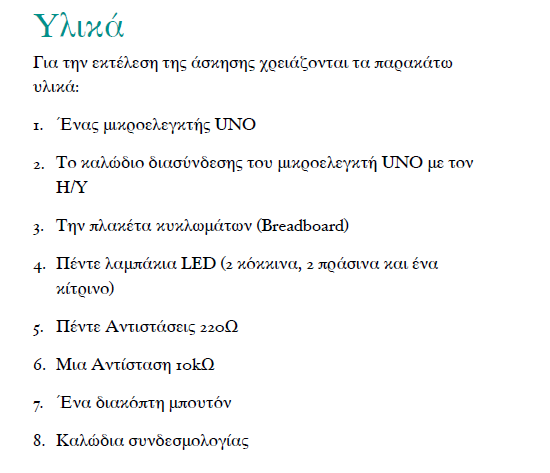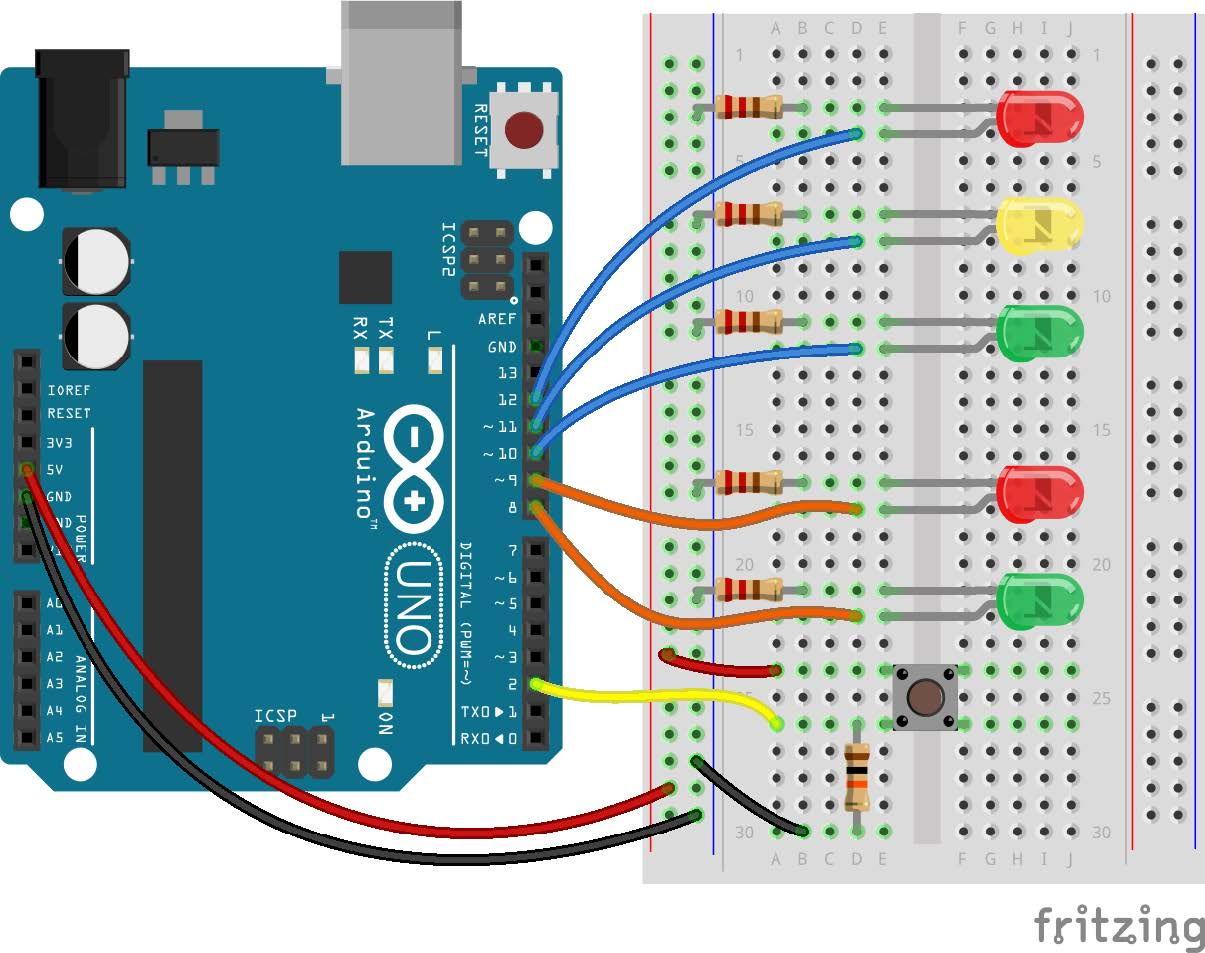tel. 24910 23755 / fax 24910 26620`/*`
`Traffic light with a pedestrian button`
`*/`
`// constants won't change. They're used here to`
`// set pin numbers:`
`// in Pin 12 we will connect the car red LED.`
`// Give it a name.`
`int carRed = 12;`
`// in Pin 11 we will connect the car yellow LED.`
`// Give it a name.`
`int carYellow = 11;`
`// in Pin 10 we will connect the car green LED.`
`// Give it a name.`
`int carGreen = 10;`
`// in Pin 9 we will connect the pedestrian red`
`// LED. Give it a name.`
`int pedRed = 9; //ped lights being assigned`
`// in Pin 8 we will connect the pedestrian green`
`// LED. Give it a name.`
`int pedGreen = 8;`
`// in Pin 12 we will connect the pedestrian`
`// button. Give it a name.`
`int button = 2;`
`//crossing time given to pedestrians`
`int crossTime = 5000;`
`// Variables`
`// collects the time since the button was last`
`// pressed`
`unsigned long changeTime;`
`// store pedestrian press button`
`int pedestrian;`
`// the setup routine runs once when you press`
`// reset:`
`void setup() {`
`  // initialize the digital pin as an output.`
`  pinMode(carRed, OUTPUT);`
`  pinMode(carYellow, OUTPUT);`
`  pinMode(carGreen, OUTPUT);`
`  pinMode(pedRed, OUTPUT);`
`  pinMode(pedGreen, OUTPUT);`
`  // initialize the digital pin as an input`
`  pinMode(button, INPUT);`
`  // start with green car light on`
`  digitalWrite(carGreen,HIGH);`
`  // start with red ped light on`
`  digitalWrite(pedRed, HIGH);`
`  // initialize changeTime`
`  changeTime = millis();`
`  // initialize preState`
`  pedestrian = LOW;`
`}`
`// the loop routine runs over and over again`
`// forever:`
`void loop() {`
`  // check if button is pressed`
`  // and it is over 5 sec since last button`
`  // press`
`  int state = digitalRead(button);`
`  if (state == HIGH) {`
`  pedestrian = HIGH;`
`  }`
`  if (pedestrian == HIGH &&`
`   (millis() - changeTime) > 15000) {`
`   //function to change lights`
`   changeLights();`
`   changeTime = millis();`
`   pedestrian = LOW;`
`  }`
`}`
`void changeLights() {`
`  //green car light off`
`  digitalWrite(carGreen,LOW);`
`  // yellow car light on`
`  digitalWrite(carYellow,HIGH);`
`  //wait 2 seconds`
`  delay(2000);`
`  // yellow car light off`
`  digitalWrite(carYellow,LOW);`
`  //red car light on`
`  digitalWrite(carRed,HIGH);`
`  //wait 1 second to turn on ped light`
`  delay(1000);`
`  //red ped light off`
`  digitalWrite(pedRed,LOW);`
`  //green ped light on. allow crossing`
`  digitalWrite(pedGreen,HIGH);`
`  //delay preset time of 5 seconds`
`  delay(crossTime);`
`  //flashing of ped green light`
`  for (int x=0; x<10; x++) {`
`   digitalWrite(pedGreen,HIGH);`
`   delay(250);`
`   digitalWrite(pedGreen,LOW);`
`   delay(250);`
`  }`
`  //turn red ped light on`
`  digitalWrite(pedRed, HIGH);`
`  delay(100);`
`  //car green light on`
`  digitalWrite(carGreen,HIGH);`
`  //car red light off`
`  digitalWrite(carRed,LOW);`
`}`

Κατηγορίες: Arduino